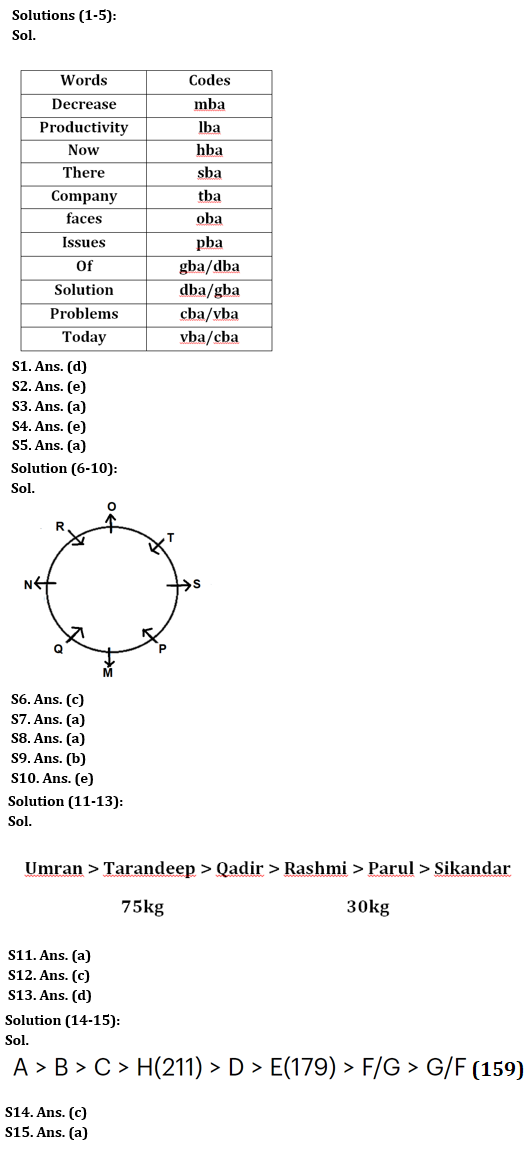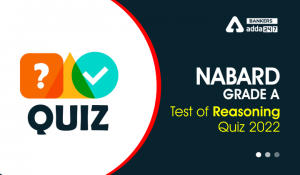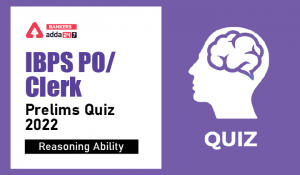Latest Banking jobs   »

# Reasoning Ability Quiz For IBPS RRB PO Prelims 2022- 4th July

Directions (1-5): Answer the questions based on the information given below.
In a certain language,
‘Decrease productivity now there’ is coded as ‘Iba sba mba hba’
‘Company there faces issue’ is coded as ‘oba sba tba pba’
‘Decrease issue of solution’ is coded as ‘dba pba mba gba’
‘Productivity faces problems today’ is coded as ‘vba Iba cba oba’

Q1. Which of the following is the code for ‘company’ in the given language?
(a) dba
(b) pba
(c) oba
(d) tba
(e) None of these

Q2. Which of the following words is coded as ‘gba’ in the given language?
(a) Of
(b) Situation
(c) Now
(d) There
(e) Either ‘of’ or ‘situation’

Q3. Which of the following is coded as ‘sba’?
(a) There
(b) Now
(c) Productivity
(d) Either ‘there’ or ‘now’
(e) Of

Q4. What is the code of the words ‘Productivity today’?
(a) hba vba
(b) lba vba
(c) Iba cba
(d) cba hba
(e) Either (b) or (c)

Q5. What is the code of the word ‘Issue’?
(a) pba
(b) hba
(c) tba
(d) lba
(e) None of these

Directions (6-10): Answer the following question based on the information given below:
Eight people M, N, O, P, Q, R, S and T are sitting around a circular table, such that four of them are facing inside and four of them are facing outside. No two adjacent people are facing in the same direction. (For example: if P and N are sitting adjacent to each other and P is facing inside then N must be facing outside).
N is sitting second to the right of M. Three people are sitting between P and R. M is not sitting adjacent to R. O is sitting third to the right of P. Number of people sitting between S and R is more than three, when counted from the right of R. S is sitting immediate left of T. S is not sitting opposite to Q. Q is facing inside.

Q6. How many people are sitting between P and O when counted from the left of O?
(a) Two
(b) Three
(c) Four
(d) Five
(e) One

Q7. Who is sitting to the immediate right of S?
(a) P
(b) M
(c) N
(d) O
(e) R

Q8. Four of the following five are related to each other in some way and thus form a group. Choose the one which does not belong to that group.
(a) N
(b) T
(c) R
(d) P
(e) Q

Q9. What is the position of P with respect to T?
(a) Second to the right
(b) Second to the left
(c) Third to the right
(d) Third to the left
(e) Sixth to the left

Q10. Who is sitting to the immediate right of N?
(a) P
(b) M
(c) Q
(d) O
(e) R

Directions (11-13): Answer the questions based on the information given below.
Six persons have different weights. Qadir is heavier than Parul but lighter than Tarandeep. The one whose weight is 30 kg, is the second lightest person while the one whose weight is 75 kg, is the second heaviest person. Sikandar is just lighter than Parul. Rashmi is lighter than Umran. The weight of Rashmi is not 75 kg. Tarandeep is not the heaviest. Qadir is heavier than Rashmi who is not the lightest.

Q11. Who among the following is the 3rd lightest person?
(a) Rashmi
(b) Parul
(d) Umran
(e) None of the above

Q12. Who among the following is the 3rd heaviest person?
(a) Rashmi
(b) Parul
(d) Umran
(e) None of the above

Q13. If the weight of Qadir is 25kg more than the weight of Parul, then what could be the weight of Rashmi?
(a) 56
(b) 60
(c) 65
(d) 50
(e) 29

Directions (14-15): Answer the following question based on the information given below:
There are eight students A, B, C, D, E, F, G and H in a class. Each of them scored different marks.
E scored more marks than only F and G. H scored more marks than D but less marks than C. Lowest marks scored is 159. E scored 179 marks. B scored less marks than only A. H scored 211 marks.

Q14. If G scored less than F and marks scored by F is the average of E and G, then what is the marks scored by F?
(a) 170
(b) 168
(c) 169
(d) 175
(e) None of these

Q15. What can be the marks scored by B?
(a) 215
(b) 210
(c) 178
(d) 153
(e) 147

Solutions#### Congratulations!Incorrect details? Fill the form again here

•Reasoning Ability Quiz For IBPS RRB PO C...
•Reasoning Ability Quiz For NABARD Grade ...
•Reasoning Ability Quiz For IBPS Clerk/PO...
•Reasoning Ability Quiz For IBPS RRB PO C...
•Reasoning Ability Quiz For IBPS RRB PO C...
•Reasoning Ability Quiz For IBPS Clerk Pr...